# Addition of Roman numbers

Added together and write as decimal number:

LXVII + MLXIV

Result

LXVII + MLXIV =  1131

#### Solution:Leave us a comment of example and its solution (i.e. if it is still somewhat unclear...):Be the first to comment!## Next similar examples:

1. Valid numberRound the 453874528 on 2 significant numbers.
2. Foot in busIt was 102 people on the bus. 28 girls had two dogs. A 11 girls had one dog. At the next stop seceded 5 dogs (even with their owners). They got two boys together with three dogs. The bus drove one driver. How many foot were in bus?
3. CakesOn the bowl were a few cakes. Jane ate one-third of them, Dana ate a quarter of those cakes that remained. a) What part (of the original number of cakes) Dana ate? b) At least how many cakes could be (initially) on thebowl?
4. The resultHow many times I decrease the number 1632 to get the result 24?
5. Flood waterFlood waters in some US village meant that the homes had to evacuate 364 people. 50 of them stayed at elementary schools, 59 them slept with their friends and others went to relatives. How many people have gone to relatives?
6. If-then equationIf 5x - 17 = -x + 7, then x =
7. Math classificationIn 3A class are 27 students. One-third got a B in math and the rest got A. How many students received a B in math?
8. Six te 2If 3t-7=5t, then 6t=
9. One frame5 picture frames cost € 12 more than three frames. How much cost one frame?
10. Expressions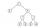Find out value of expressions if a = -1, b =2: x=b - 2a - ab y=a3 - b2 - 2ab z=a2 b3 - a3 b2 w=a + b + a3 - b2
11. Plane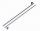On how many parts divide plane 6 parallels?
12. Line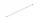How many parts of line divide 5 (different) points that lie on it?
13. Ten pupils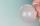10 pupils went to the store. 6 pupils bought lollipops and 9 pupils bought chewing gum. How many pupils have bought both lollipops and chewing gums (if everyone bought something)?
14. Eq1Solve equation: 4(a-3)=3(2a-5)
15. CagesHonza had three cages (black, silver, gold) and three animals (guinea pig, rat and puppy). There was one animal in each cage. The golden cage stood to the left of the black cage. The silver cage stood on the right of the guinea pig cage. The rat was in the
16. Circles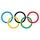For the circle c1(S1; r1=146 cm) and c2(S2; r2 = 144 cm) is distance of centers |S1S2| = 295 cm. Determine the distance between the circles.
17. Angles 1It is true neighboring angles have not common arm?